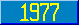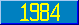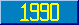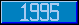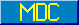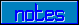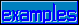## Numeric Data Values

### 7.1.4.3 Numeric data values

All variables, local, global, and special, have values which are either defined or undefined. If defined, the values may always be thought of and operated upon as strings. The set of numeric values is a subset of the set of all data values.

Only numbers which may be represented with a finite number of decimal digits are representable as numeric values. A data value has the form of a number if it satisfies the following restrictions.

1. It shall contain only digits and the characters "-" and ".".
2. At least one digit must be present.
3. "." occurs at most once.
4. The number zero is represented by the one-character string "0".
5. The representation of each positive number contains no "-".
6. The representation of each negative number contains the character "-" followed by the representation of the positive number which is the absolute value of the negative number. (Thus, the following restrictions describe positive numbers only.)
7. The representation of each positive integer contains only digits and no leading zero.
8. The representation of each positive number less than 1 consists of a "." followed by a nonempty digit string with no trailing zero. (This is called a fraction.)
9. The representation of each positive non-integer greater than 1 consists of the representation of a positive integer (called the integer part of the number) followed by a fraction (called the fraction part of the number).

Note that the mapping between representable numbers and representations is one-to-one. An important result of this is that string equality of numeric values is a necessary and sufficient condition of numeric equality.